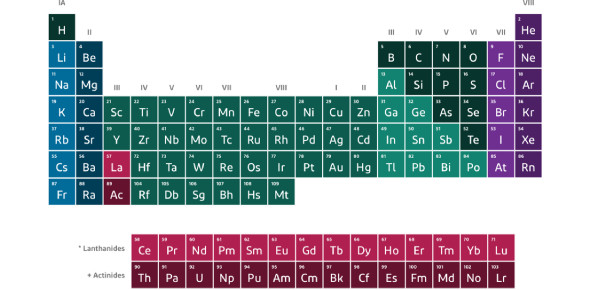# Trivia On Periodic Table: Quiz!

10 Questions | Total Attempts: 4044Settings.

• 1.
Which of the following elements has the most protons?
• A.

• B.

• C.

Zinc

• D.

Bromine

• E.

Mercury

• 2.
Which of the following elements has the largest atoms?
• A.

Oxygen

• B.

Sulfur

• C.

Selenium

• D.

Tellurium

• E.

Polonium

• 3.
Why does the size of an atom decrease as you move to the right across rows on the periodic table?
• A.

The have more protons (which results in more pull toward the center).

• B.

They have more neutrons (which make the atom more compact).

• C.

They have more electrons (which increase the size of the atom).

• D.

They have less electrons (because they tend to lose electrons).

• E.

They have less neutrons (which give them a positive charge).

• 4.
Which of the following is the largest particle?
• A.

Ca^2+

• B.

Ca^1+

• C.

Ca

• D.

Ca^1-

• E.

Ca^2-

• 5.
Which of the following is smaller?
• A.

Lithium as a cation

• B.

Lithium metal

• C.

Lithium anion

• 6.
Which word represents the amount that an atom wants electrons when it is in a molecule?
• A.

Electron Affinity

• B.

• C.

Electronegativity

• D.

Ionization Energy

• 7.
Why does fluorine have a high ionization energy?
• A.

It has a low number of shells and a low number of protons.

• B.

It has a low number of shells and a high number of protons.

• C.

It has a high number of shells and a low number of protons.

• D.

It has a high number of shells and a high number of protons.

• 8.
Who is considered the "father of the periodic table"?
• A.

Mosely

• B.

Mendeleev

• C.

Rutherford

• D.

Einstein

• 9.
The periodic table is arranged in order of increasing.
• A.

Atomic mass

• B.

Number of neutrons

• C.

Atomic number

• D.

Reactivity

• 10.
Why does ionization energy decrease as you move down a group on the periodic table?
• A.

The electrons are farther from the nucleus making it more difficult to remove.

• B.

The electrons are farther from the nucleus making it easier to remove.

• C.

The electrons are closer to the nucleus making it more difficult to remove.

• D.

The electrons are closer to the nucleus making it easier to remove.

Related TopicsBack to top